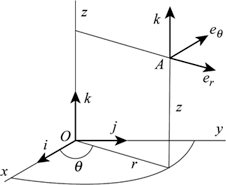# A triangle 6

A triangle has vertices on a coordinate grid at H(-2,7), I(4,7), and J(4,-9). What is the length, in units, of vector HI?

h =  6

### Step-by-step explanation:Did you find an error or inaccuracy? Feel free to write us. Thank you!

Tips for related online calculators
Our vector sum calculator can add two vectors given by their magnitudes and by included angle.
Do you want to convert length units?
The Pythagorean theorem is the base for the right triangle calculator.